# Debt Schedule

Learn the Steps to Build a Debt Schedule in Excel• What is the purpose behind modeling a debt schedule?
• Why is issuing payments to lenders in order of priority such an important matter?
• What is the difference between mandatory principal amortization and cash sweep?
• How is interest expense for each debt tranche calculated?

## Debt Schedule Modeling Overview

The purpose behind modeling the debt schedule is to forecast the balances of outstanding debt securities and the amount of interest expense coming due in each period.

For a company raising debt financing, it is essential to determine the impact of the new debt on its free cash flows (FCFs) and credit metrics.

The parties involved in a lending arrangement – or more specifically, the borrower and lender(s) – are entering a contractual legal agreement. In exchange for capital from the lender(s), the borrowers agree to terms such as:

• Interest Expense – The cost of borrowing debt capital – i.e. the amount charged by the lender to the borrower throughout the term of the debt (i.e. the borrowing period).
• Mandatory Amortization – Typically associated with senior lenders, amortization is the required incremental paydown of the debt principal throughout the lending term.
• Principal Repayment – On the date of maturity, the original principal amount must be repaid in full (i.e. a “bullet” lump-sum payment).

Loan agreements are legally-binding contracts with specific requirements that must be followed. For example, paying a lender with lower priority ahead of a senior lender is a clear violation unless explicit approval was provided.

If a company defaults on a debt obligation and undergoes liquidation, the seniority of each creditor determines the order in which lenders would receive proceeds (i.e. recovery).

## Senior Debt vs Subordinated Debt

The required rate of return is higher for non-senior lenders lower in the capital structure as these lenders require more compensation for undertaking incremental risk.

The two distinct types of debt structures are as follows.

1. Senior Debt – e.g. Revolver, Term Loans
2. Subordinated Debt – e.g. Investment-Grade Bonds, Speculative-Grade Bonds (High-Yield Bonds, or “HYBs”), Convertible Bonds, Mezzanine Securities

Senior debt lenders such as banks tend to be more risk-averse while prioritizing capital preservation (i.e. downside protection), whereas subordinated debt investors are typically more yield-oriented.

The revolving credit facility – i.e. the “revolver” – is a flexible form of short-term financing in which the borrower can draw down (i.e. obtain more debt) or repay as needed once the borrower has enough cash.

However, if the borrower has an outstanding revolver balance, all discretionary debt repayments must go towards paying down the revolver balance.

There are two major aspects in a typical credit agreement that reduce the outstanding debt over time:

1. Mandatory Amortization – The required repayment of some amount of the original debt principal, typically meant to de-risk the lender’s investment over time.
2. Optional Cash Sweep – The discretionary decision by a company to repay more debt principal ahead of schedule; albeit there are often fines for early pre-payment.

## Debt Schedule Excel Template

Now that we’ve listed out the steps to building out a debt schedule, we can move on to an example modeling exercise in Excel. For access to the template, fill out the form below: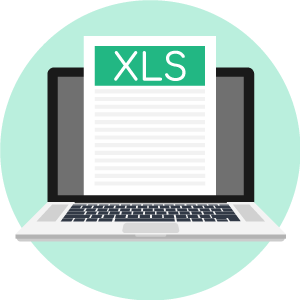Submitting ...

## Debt Financing Assumptions

The first step to modeling a debt schedule is to create a table outlining each of the different tranches of debt along with their respective lending terms.

Here, our company has three different tranches of debt within its capital structure:

1. Revolving Credit Facility (i.e. Revolver)
2. Senior Debt
3. Subordinated Debt

In the first column (D), we have “x EBITDA”, which refers to how much debt was raised in that particular tranche relative to EBITDA – i.e. “turns” of EBITDA.

For the sake of simplicity, we’ll be referencing our next twelve months (NTM) EBITDA figure for our debt amounts.

For instance, our company raised 3.0x EBITDA, so we multiply our Year 1 EBITDA of \$100m – i.e. the next fiscal year – by 3.0x to get \$300m in senior debt capital.

• Revolver = 0.0x * \$100m EBITDA = \$0m
• Senior Debt = 3.0x * \$100m EBITDA = \$300m
• Subordinated Debt = 1.0x * \$100m EBITDA = \$100m

Since the total leverage multiple is 4.0x, the total amount of debt is \$400m.

• Total Debt = \$300m Senior Debt + \$100m Subordinated Debt = \$400m Total Debt

## Debt Interest Rate Pricing

The next two columns following the “\$ Amount” section are “Pricing” and “% Floor”, which we’ll be using the determine the interest expense burden associated with each debt tranche.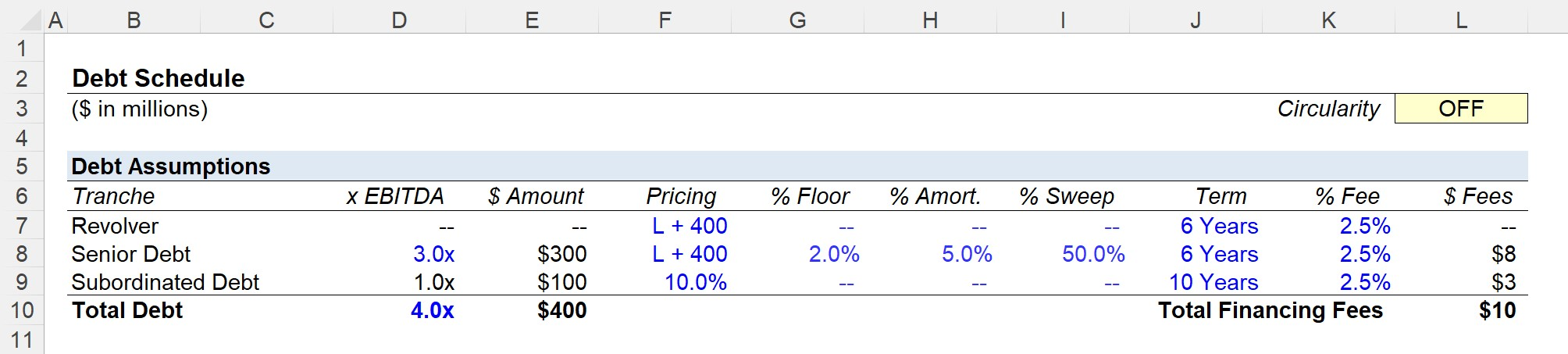For the revolver, the pricing is “LIBOR + 400”, which means the interest expense is the rate of LIBOR plus 400 basis points (bps) – i.e. one-hundredth of a percent.

With that said, to convert basis points to percentage form, we just divide by 10,000.

• Revolver Interest Rate = 1.2% + 4.0% = 5.2%

For the senior debt tranche, there is an interest rate “floor”, which protects the lenders from falling interest rates (and their yield).

Our formula uses the “MAX” function in Excel to ensure that LIBOR does NOT fall below 2.0% (or 200 basis points).

If LIBOR does indeed drop below 200 bps, the interest rate is calculated as follows.

• Senior Debt Interest Rate = 2.0% + 4.0% = 6.0%

Note that LIBOR is currently in the process of being phased out by the end of 2021.

When it comes to interest rate pricing, floating interest rates are more common for senior debt than subordinated debt.

For sub-debt, a fixed rate is far more common – with the occasional PIK interest element for riskier securities or deals with a substantial amount of debt involved.

• Subordinated Debt Interest Rate = 10.0%

## Mandatory Repayment

The “% Amort.” column refers to the required repayment of the debt principal per the original lending agreement – for our scenario, this is applicable for just the senior debt (i.e. 5% annual mandatory amortization).

When modeling out the mandatory amortization, two important considerations to keep in mind are:

1. The mandatory repayment is based on the original principal amount, not the beginning balance
2. The ending debt balance cannot dip below zero, as that would mean that the borrower paid back more than the initial principal owed.

The Excel formula for mandatory repayment is as follows:

• Mandatory Repayment = -MIN (Original Principal * % Amortization, Original Principal)

## Financing Fees

The financing fees are the costs associated with raising debt capital, which are not treated as a one-time outflow but are instead expensed on the income statement under accrual accounting as a result of the matching principle.

To calculate the total financing fees, we multiply each % fee assumption by the amount raised in each tranche and then add them all up.

But to calculate the annualized financing fees, which is the amount expensed on the income statement and what impacts the free cash flow (FCF), we divide each total amount of fees in the debt tranche by the term length.

## Optional Repayment

If our company has excess cash on hand and the lending terms do not restrict early repayment, the borrower can use the excess cash for discretionary debt repayments ahead of the original schedule – which is a feature often called a “cash sweep.”

The formula for modeling the optional repayment line is:

• Optional Repayment = -MIN (SUM of Beginning Balance and Mandatory Repayment), Cash Available for Optional Paydown) * % Cash Sweep

In our illustrative example, the only tranche with the optional cash sweep feature is the senior debt, which we entered as 50% in our debt assumptions earlier.

This means that half (50%) of the discretionary, excess FCF of the company is used to repay outstanding senior debt.

## Financial Forecast

Next, for the financial forecast, we’ll use the following operating assumptions.

###### Operating Assumptions
• EBITDA = \$100m in Year 1 – Increase by +\$5m / Year
• Tax Rate = 30.0%
• D&A and CapEx = \$10m / Year
• Increase in NWC = -\$2m / Year
• Beginning Cash Balance = \$50m

Once we calculate the free cash flow (FCF) up until the point at which “Mandatory Debt Repayment” is to be paid, we add up each of the mandatory amortization amounts and link it back to our financial forecast section.

From the total amount of free cash flow available to pay down debt, we first subtract the mandatory amortization amount.

• Positive Balance – If the company has “excess cash” to service more debt, it can use the excess funds for optional repayment of debt prior to the maturity date – i.e. the “cash sweep” – or pay down the outstanding revolver balance, if applicable. The company may also retain any excess cash.
• Negative Balance – If the FCF amount is negative, the company has insufficient cash and must draw down on its revolver (i.e. borrow cash from the credit line).

For example, if we track the flow of funds in Year 1, the following changes occur:

• Free Cash Flow (Pre-Debt Repayment) = \$42m
• Less: \$15m in Mandatory Repayment
• Cash Available for Revolver Repayment = \$27m
• Less: \$14m in Optional Repayment
• Net Change in Cash = \$14m

The net change in cash of \$14m is then added to the beginning cash balance of \$50m to get \$64m as the ending cash balance in Year 1.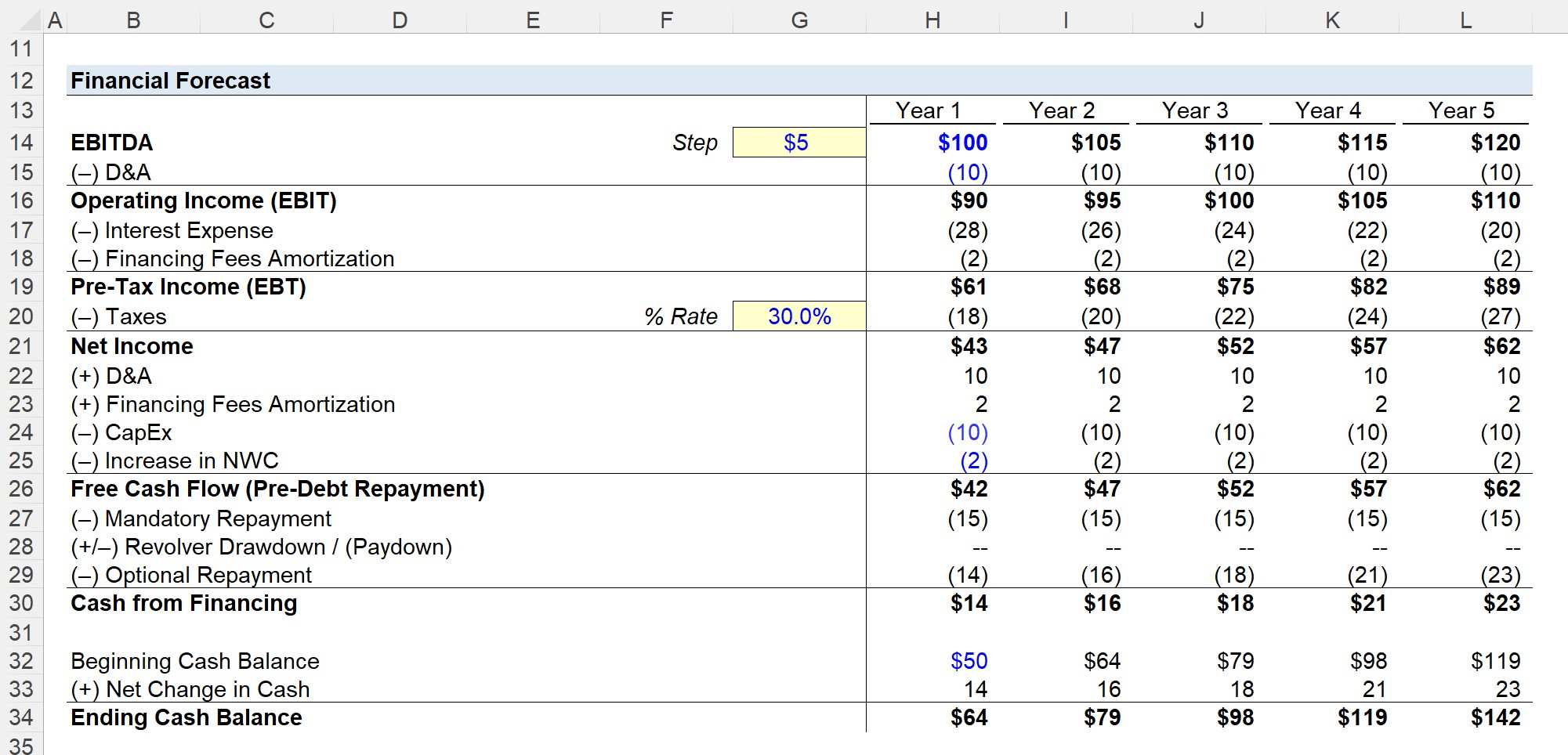## Debt Schedule Build

In the final section of our debt schedule, we’ll calculate the ending debt balances for each tranche, as well as the total interest expense.

1. Calculating the total debt balance is straightforward, as you just add up the ending balances of each tranche for each period.
2. Interest expense is calculated using the average debt balances – i.e. the average between the beginning and ending balance.

But prior to doing so, we must link back the missing section of the financial forecast into our debt schedule section, as shown below in the roll-forward schedules for each tranche of debt.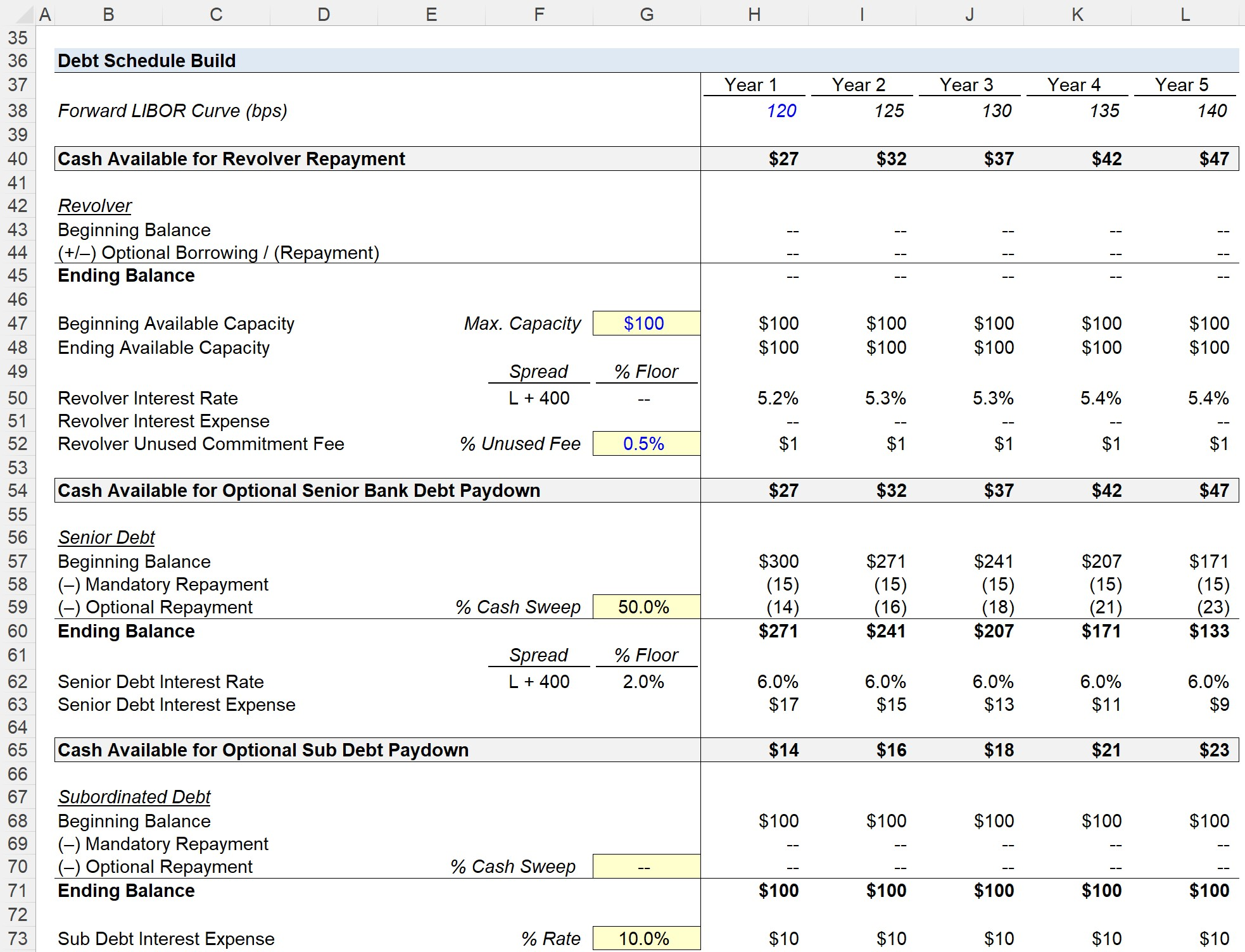Note that a circular reference is introduced into our model since interest expense reduces net income and net income reduces the free cash flow (FCF) available for debt repayment. And then, FCF affects the end-of-period debt balances and thus the interest expense for each period.

As a result, we must create a circuit breaker (i.e. the cell named “Circ”), which is a toggle switch that can cut off the circularity in the case of errors.

If the circuit breaker is set to “1”, the average balance is used in the interest expense calculation, whereas if the circuit breaker is switched to “0”, the formula will output zero in the interest expense calculations.

From Year 1 to Year 5, we can see how the total debt outstanding has declined from \$371m to \$233m, so the ending debt outstanding at the end of the projection period is 58.2% of the initial amount of debt raised.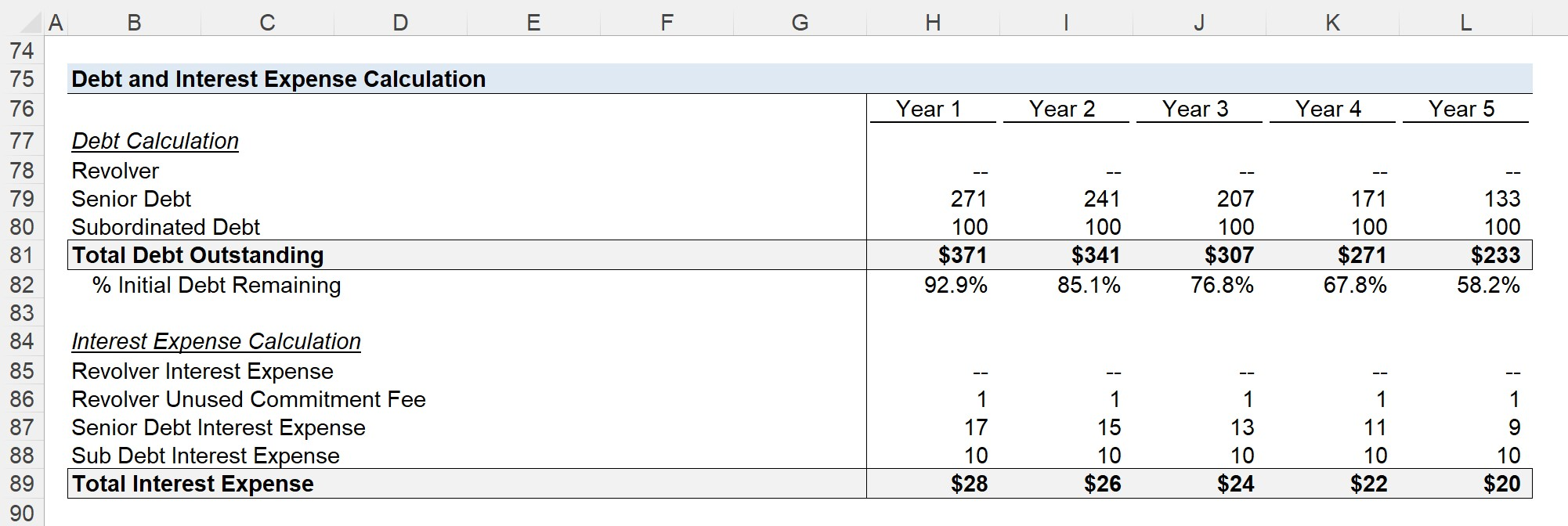Step-by-Step Online Course

#### Everything You Need To Master Financial Modeling

Enroll in The Premium Package: Learn Financial Statement Modeling, DCF, M&A, LBO and Comps. The same training program used at top investment banks.Inline FeedbacksLearn Financial Modeling Online

Everything you need to master financial and valuation modeling: 3-Statement Modeling, DCF, Comps, M&A and LBO.

X

The Wall Street Prep Quicklesson Series

7 Free Financial Modeling Lessons

Get instant access to video lessons taught by experienced investment bankers. Learn financial statement modeling, DCF, M&A, LBO, Comps and Excel shortcuts.